# 微信跳一跳⌗

2017 年 12 月 28 日下午，微信发布了 6.6.1 版本，加入了「小游戏」功能，并提供了官方 DEMO「跳一跳」。这是一个 2.5D 插画风格的益智游戏，玩家可以通过按压屏幕时间的长短来控制这个「小人」跳跃的距离。分数越高，那么在好友排行榜更加靠前。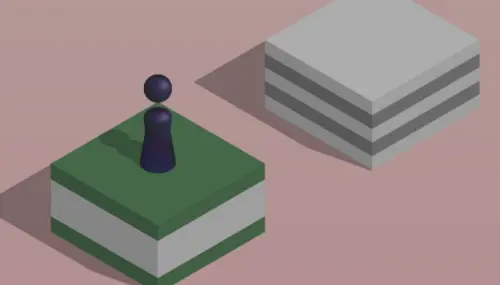# 辅助工作原理⌗

• 将手机点击到《跳一跳》小程序界面
``````    adb shell screencap -p /sdcard/autojump.webp
``````

• 手动版：用 Matplotlib 显示截图，用鼠标先点击起始点位置，然后点击目标位置，计算像素距离；

• 自动版：靠棋子的颜色来 识别棋子，靠底色和方块的色差来识别棋盘；

``````    adb shell input swipe x y x y time(ms)
``````

# 思路以及代码分析⌗

• 获取手机分辨率
``````def _get_screen_size():
'''
获取手机屏幕大小
'''
if not size_str:
sys.exit()
m = re.search(r'(\\d+)x(\\d+)', size_str)
if m:
return "{height}x{width}".format(height=m.group(2), width=m.group(1))
return "1920x1080"

``````

• 调用配置文件
``````def open_accordant_config():
'''
调用配置文件
'''
screen_size = _get_screen_size()
config_file = "{path}/config/{screen_size}/config.json".format(
path=sys.path,
screen_size=screen_size
)
if os.path.exists(config_file):
with open(config_file, 'r') as f:
else:
with open('{}/config/default.json'.format(sys.path), 'r') as f:
``````

``````if screenshot_way == 2 or screenshot_way == 1:
process = subprocess.Popen('adb shell screencap -p', shell=True, stdout=subprocess.PIPE)
if screenshot_way == 2:
binary_screenshot = screenshot.replace(b'\r\n', b'\n')
else:
binary_screenshot = screenshot.replace(b'\r\r\n', b'\n')
f = open('autojump.webp', 'wb')
f.write(binary_screenshot)
f.close()
``````

3.关键步骤：寻找棋子坐标和下一跳棋盘坐标

• 识别棋子：靠棋子的颜色来识别位置，通过截图发现最下面一行大概是一条直线，就从上往下一行一行遍历，比较颜色（颜色用了一个区间来比较）找到最下面的那一行的所有点，然后求个中点，求好之后再让 Y 轴坐标减小棋子底盘的一半高度从而得到中心点的坐标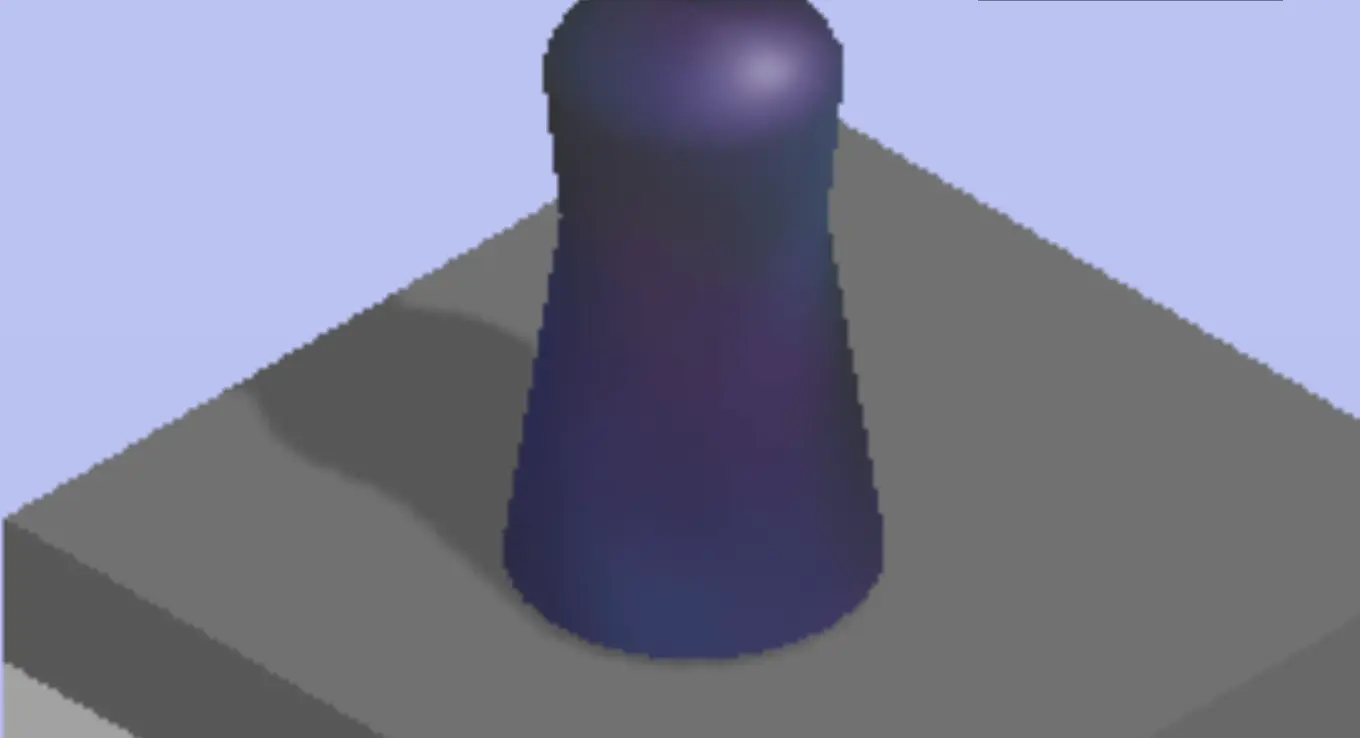``````def find_piece_and_board(im):
'''
寻找关键坐标
'''
w, h = im.size

piece_x_sum = 0
piece_x_c = 0
piece_y_max = 0
board_x = 0
board_y = 0
scan_x_border = int(w / 8)  # 扫描棋子时的左右边界
scan_start_y = 0  # 扫描的起始 y 坐标
# 以 50px 步长，尝试探测 scan_start_y
for i in range(int(h / 3), int(h*2 / 3), 50):
last_pixel = im_pixel[0, i]
for j in range(1, w):
pixel = im_pixel[j, i]
# 不是纯色的线，则记录 scan_start_y 的值，准备跳出循环
if pixel != last_pixel or pixel != last_pixel or pixel != last_pixel:
scan_start_y = i - 50
break
if scan_start_y:
break
print('scan_start_y: {}'.format(scan_start_y))

# 从 scan_start_y 开始往下扫描，棋子应位于屏幕上半部分，这里暂定不超过 2/3
for i in range(scan_start_y, int(h * 2 / 3)):
for j in range(scan_x_border, w - scan_x_border):  # 横坐标方面也减少了一部分扫描开销
pixel = im_pixel[j, i]
# 根据棋子的最低行的颜色判断，找最后一行那些点的平均值，这个颜色这样应该 OK，暂时不提出来
if (50 < pixel < 60) and (53 < pixel < 63) and (95 < pixel < 110):
piece_x_sum += j
piece_x_c += 1
piece_y_max = max(i, piece_y_max)

if not all((piece_x_sum, piece_x_c)):
return 0, 0, 0, 0
piece_x = int(piece_x_sum / piece_x_c)
piece_y = piece_y_max - piece_base_height_1_2  # 上移棋子底盘高度的一半
``````

• 识别棋盘
``````if piece_x < w/2:
board_x_start = piece_x
board_x_end = w
else:
board_x_start = 0
board_x_end = piece_x
``````

``````   for i in range(int(h / 3), int(h * 2 / 3)):
last_pixel = im_pixel[0, i]
if board_x or board_y:
break
board_x_sum = 0
board_x_c = 0

for j in range(int(board_x_start), int(board_x_end)):
pixel = im_pixel[j, i]
# 修掉脑袋比下一个小格子还高的情况的 bug
if abs(j - piece_x) < piece_body_width:
continue

# 修掉圆顶的时候一条线导致的小 bug，这个颜色判断应该 OK，暂时不提出来
if abs(pixel - last_pixel) + abs(pixel - last_pixel) + abs(pixel - last_pixel) > 10:
board_x_sum += j
board_x_c += 1
if board_x_sum:
board_x = board_x_sum / board_x_c
last_pixel = im_pixel[board_x, i]

# 从上顶点往下 +274 的位置开始向上找颜色与上顶点一样的点，为下顶点
# 该方法对所有纯色平面和部分非纯色平面有效，对高尔夫草坪面、木纹桌面、药瓶和非菱形的碟机（好像是）会判断错误
for k in range(i+274, i, -1): # 274 取开局时最大的方块的上下顶点距离
pixel = im_pixel[board_x, k]
if abs(pixel - last_pixel) + abs(pixel - last_pixel) + abs(pixel - last_pixel) < 10:
break
board_y = int((i+k) / 2)

# 如果上一跳命中中间，则下个目标中心会出现 r245 g245 b245 的点，利用这个属性弥补上一段代码可能存在的判断错误
# 若上一跳由于某种原因没有跳到正中间，而下一跳恰好有无法正确识别花纹，则有可能游戏失败，由于花纹面积通常比较大，失败概率较低
for l in range(i, i+200):
pixel = im_pixel[board_x, l]
if abs(pixel - 245) + abs(pixel - 245) + abs(pixel - 245) == 0:
board_y = l+10
break

if not all((board_x, board_y)):
return 0, 0, 0, 0

return piece_x, piece_y, board_x, board_y
``````

274像素的寻找方式：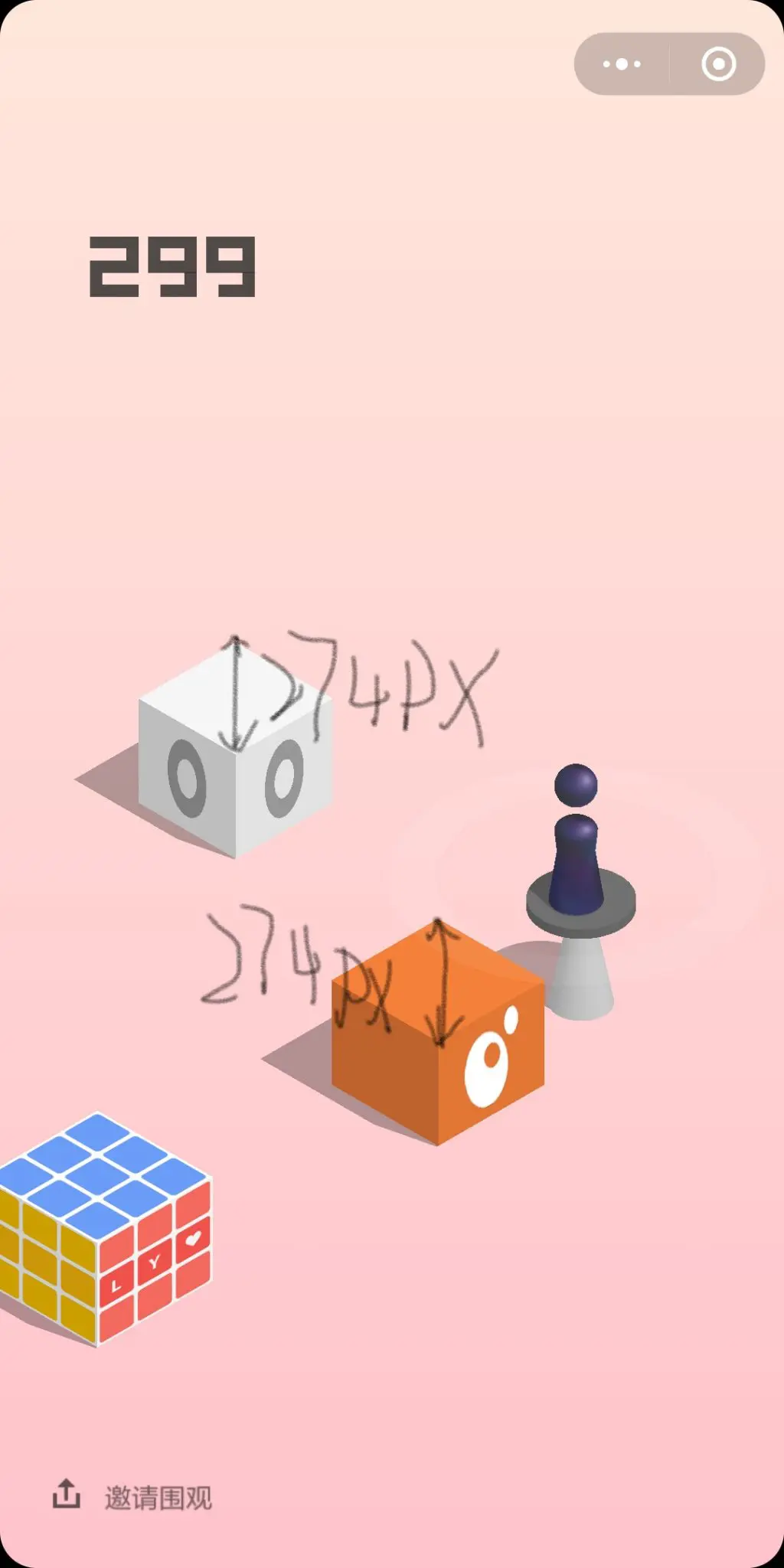4.测算两点距离和点按时间确定

• 两点距离计算 距离的求法很简单，和初中直角坐标系下两点距离公式一致：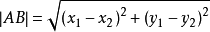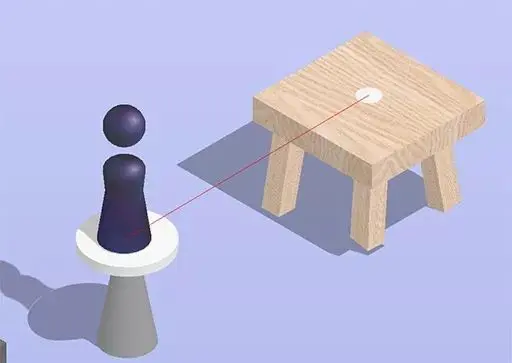``````math.sqrt((board_x - piece_x) ** 2 + (board_y - piece_y) ** 2)
``````
• 通过距离推算按压时间
``````def jump(distance):
'''
跳跃一定的距离
'''
press_time = distance * press_coefficient
press_time = max(press_time, 200)   # 设置 200ms 是最小的按压时间
press_time = int(press_time)
``````

``````{
"under_game_score_y": 300,
"press_coefficient": 1.392,
"piece_base_height_1_2": 20,
"piece_body_width": 70,
"swipe": {
"x1": 500,
"y1": 1600,
"x2": 500,
"y2": 1602
}
}
``````

``````adb shell input swipe x y x y time
``````

``````while True:
pull_screenshot()
im = Image.open('./autojump.webp')
# 获取棋子和 board 的位置
piece_x, piece_y, board_x, board_y = find_piece_and_board(im)
ts = int(time.time())
print(ts, piece_x, piece_y, board_x, board_y)
set_button_position(im)
jump(math.sqrt((board_x - piece_x) ** 2 + (board_y - piece_y) ** 2))
if debug_switch:
debug.save_debug_screenshot(ts, im, piece_x, piece_y, board_x, board_y)
debug.backup_screenshot(ts)
i += 1
if i == next_rest:
print('已经连续打了 {} 下，休息 {}s'.format(i, next_rest_time))
for j in range(next_rest_time):
sys.stdout.write('\r程序将在 {}s 后继续'.format(next_rest_time - j))
sys.stdout.flush()
time.sleep(1)
print('\n继续')
i, next_rest, next_rest_time = 0, random.randrange(30, 100), random.randrange(10, 60)
time.sleep(random.uniform(0.9, 1.2))   # 为了保证截图的时候应落稳了，多延迟一会儿，随机值防 ban
``````